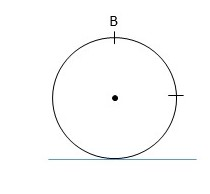# The wheel is rolling to the left with a constant angular velocity without slipping. Determine the...

## Question:

The wheel is rolling to the left with a constant angular velocity without slipping. Determine the direction of the acceleration at point B (in the regular xy-coordinate system).## Centripetal Acceleration:

Centripetal acceleration is experienced by an object undergoing circular motion. This type of acceleration is directed towards the center of the circle and is responsible for the continuous curvature of the path.

At any time, the centripetal acceleration of point B is directly towards the center of the wheel. If the wheel is not experiencing any other linear or angular acceleration, then centripetal is the only acceleration acting on B. In the configuration shown, towards the center of the circle is in the negative y-direction.Centripetal Acceleration: Definition, Formula & Example

from

Chapter 19 / Lesson 6
34K

In this lesson, you will learn the meaning of the term centripetal acceleration, an equation to calculate it, and an example of how to use the equation. A short quiz will follow.